# Problem Set 1¶

In :
import jax
import jax.numpy as np
import jax.lax as lax
import jax.scipy.stats as stats

In :
# configure matplotlib output
import matplotlib.pyplot as plt
import matplotlib as mpl
mpl.style.use('config/clean.mplstyle') # this loads my personal plotting settings
%matplotlib inline

In :
# if you have an HD display
%config InlineBackend.figure_format = 'retina'

In :
import tools.models as mod
import tools.assign as ps

WARNING:absl:No GPU/TPU found, falling back to CPU. (Set TF_CPP_MIN_LOG_LEVEL=0 and rerun for more info.)


### Setup¶

Check out assign.py, most of the implementation is hiding in there. We're just summarizing the results here. Note that assign.py uses some functions from valjax, so you'll have to have that in your Python path. Below are the parameters we'll be using. I didn't specify what to use for $\mu$, $\sigma$, and $\kappa$, but these make it so the entry distribution is well below the steady state and firms exit enough that we don't just get everyone bunched at the steady state capital level.

In :
# model parameters
par = {
'β': 0.95,
'δ': 0.1,
'α': 0.35,
'z': 1.0,
'μ': 0.5,
'σ': 0.2,
'κ': 0.1,
}

In :
# algo params
N = 1000 # capital grid size
K = 400 # number of iterations
P = 3 # number of gradient steps


# Question 1¶

Here we look at three approaches. The first is brute force grid optimization. The second is basically the same, but we also get a better estimate of the true optimum by looking at the points around the max using valjax.smoothmax. The third is using interpolation. Here we construct an objective using linear interpolation from np.interp and perform gradient updates on the policy using this. Thus in the last case the fixed point update is over the value-policy pair, rather than just the value as in the first two cases.

In :
def value_summary(vfi, ret, hist):
fig, (ax0, ax1, ax2) = plt.subplots(ncols=3, figsize=(12, 3))
ax0.plot(vfi.k_grid, ret['v']);
ax1.plot(vfi.k_grid, ret['kp']-vfi.k_grid);
ax1.scatter(vfi.kss, 0, c='k', zorder=10);
ax1.hlines(0, *ax1.get_xlim(), color='k', linestyle='--', linewidth=1);
pd.Series(hist['err']).plot(ax=ax2, logy=True)


### Grid¶

In :
vfi_grid = ps.Capital(par, N=N)
v_grid, ret_grid, hist_grid = vfi_grid.fast_solve_grid(K=K)
value_summary(vfi_grid, ret_grid, hist_grid)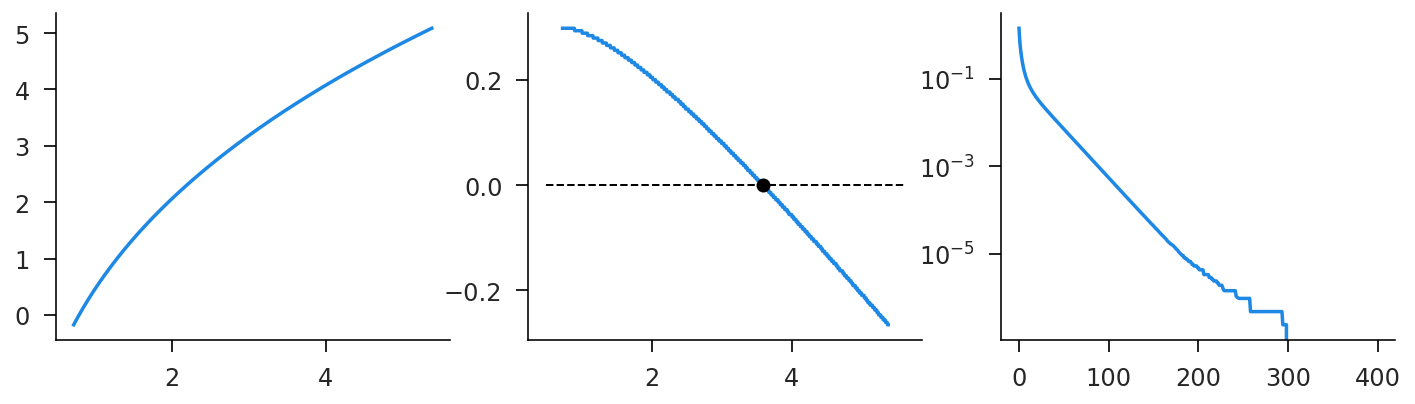### Smooth¶

In :
vfi_smooth = ps.Capital(par, smooth=True, N=N)
v_smooth, ret_smooth, hist_smooth = vfi_smooth.fast_solve_grid(K=K)
value_summary(vfi_smooth, ret_smooth, hist_smooth)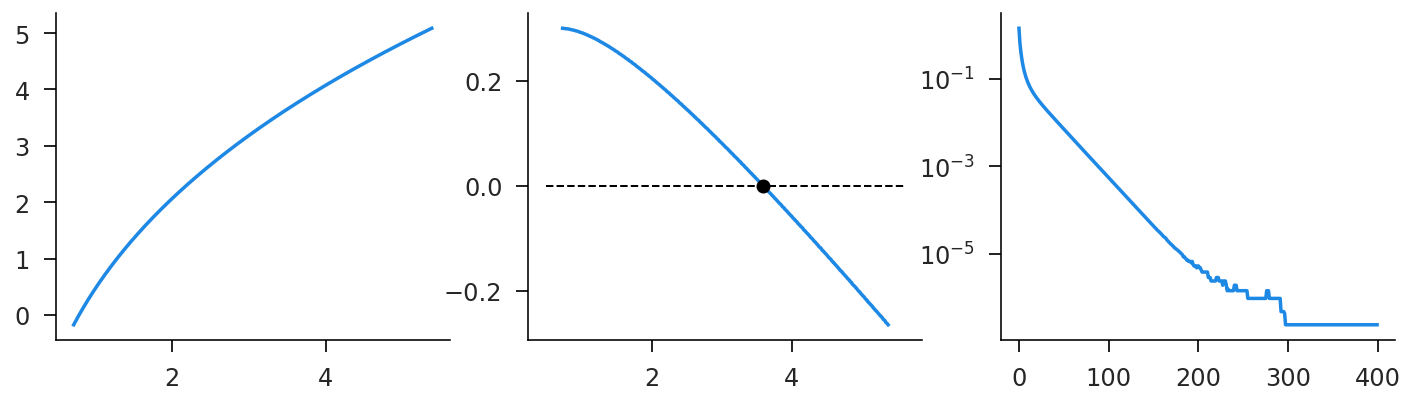### Interp¶

In :
vfi_interp = ps.Capital(par, N=N, P=P)
v_interp, kp_interp, ret_interp, hist_interp = vfi_interp.fast_solve_interp(K=K)
value_summary(vfi_interp, ret_interp, hist_interp)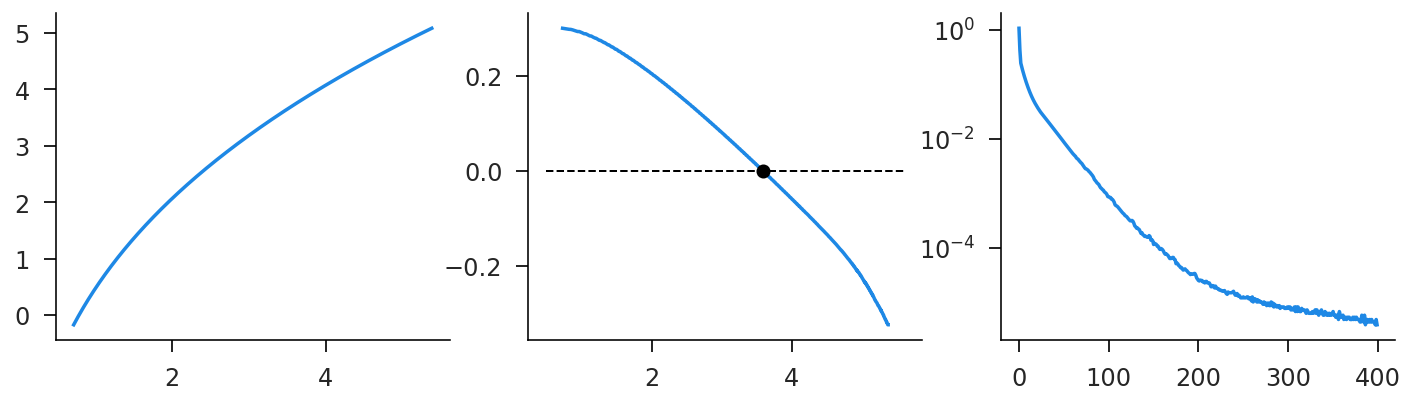### Compare¶

The timing comes out extremely similar for the first wo, which isn't surprising since they are largely doing the same computations. Interpolation is quite a bit slower. However, the memory usage is potentially much better. In both the grid cases, we construct an $N \times N$ matrix of returns to $k \times k^{\prime}$ pairs. This scales quadratically and can be prohibitive for very large state spaces. The interpolation method should scale roughly with $N$. There does appear to be some loss of numerical accuracy for the interpolation case. I suspect his is beause linear interpolation produces an objective that is not continuously differentiable. Using a higher order interpolation method might fix this.

In :
%time _ = vfi_grid.fast_solve_grid(K=K)

CPU times: user 607 ms, sys: 22.4 ms, total: 629 ms
Wall time: 585 ms

In :
%time _ = vfi_smooth.fast_solve_grid(K=K)

CPU times: user 607 ms, sys: 33.3 ms, total: 641 ms
Wall time: 578 ms

In :
%time _ = vfi_interp.fast_solve_interp(K=K)

CPU times: user 53.1 ms, sys: 4.48 ms, total: 57.6 ms
Wall time: 29.6 ms

In :
df_err = pd.DataFrame({
'grid_err': hist_grid['err'],
'smooth_err': hist_smooth['err'],
'interp_err': hist_interp['err'],
})
df_err.plot(logy=True);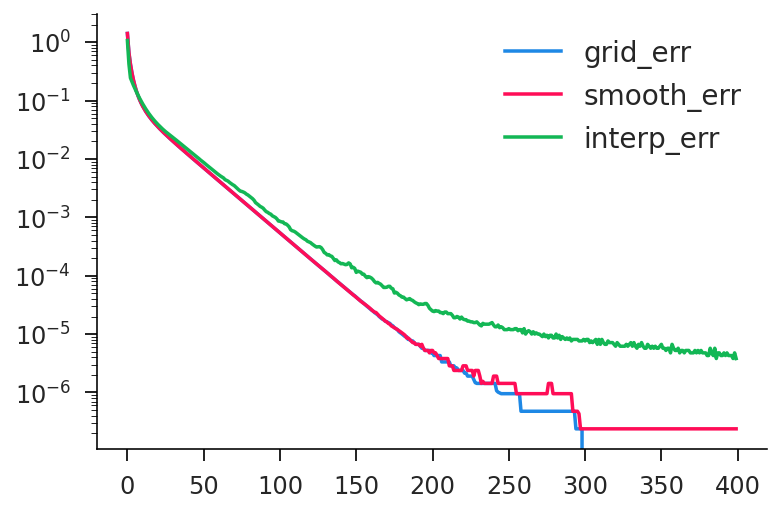In :
df_diff = pd.DataFrame({
'v_diff': np.abs(v_grid-v_interp),
'kp_diff': np.abs(ret_grid['kp']-ret_interp['kp']),
}, index=vfi_grid.k_grid)
df_diff.rolling(50).mean().plot(
logy=True, subplots=True, layout=(1, 2), figsize=(10, 4)
);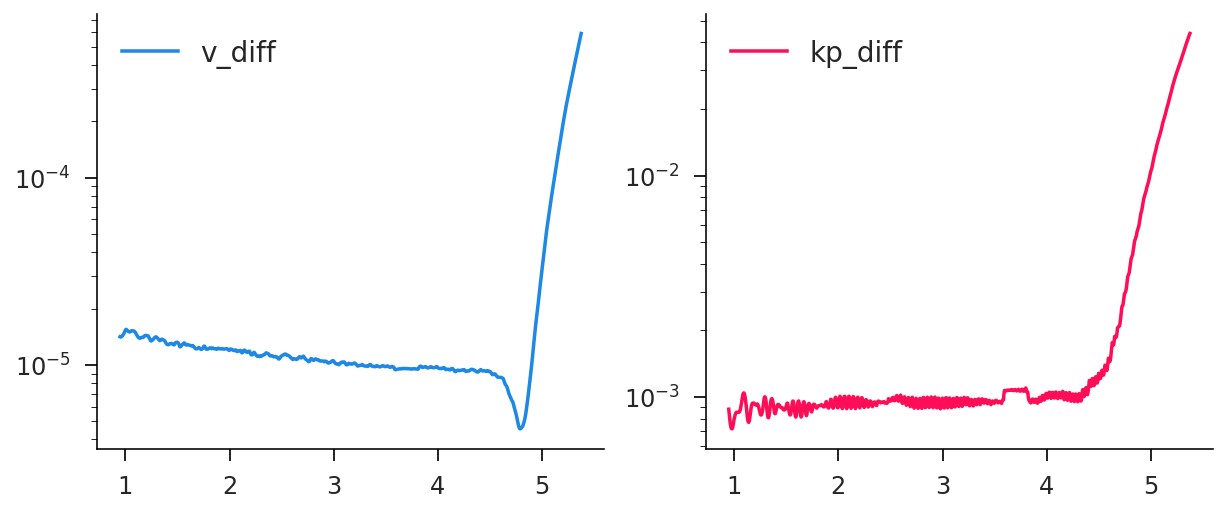# Question 2¶

Here because of the values for $\mu$ and $\sigma$, the distribution reflects entry at lower capital levels and the movement towards steady state for surviving firms. Both approaches yield the same result. But there is seemingly numerical noise that enters into the steady state distribution. I suspect that adding in some additional noise to capital levels would eliminate this.

In :
def dist_summary(vfi, dist, ax, iss=None):
iss = iss or np.argmax(dist)
ax.plot(vfi.k_grid[:iss], dist[:iss])

In :
k_dist_null = vfi_interp.fast_distribution(ret_interp['kp'], method='null')
k_dist_age = vfi_interp.fast_distribution(ret_interp['kp'], method='age')

In :
fig, (ax0, ax1) = plt.subplots(ncols=2, figsize=(10, 4))
dist_summary(vfi_interp, k_dist_null, ax0)
dist_summary(vfi_interp, k_dist_age, ax1);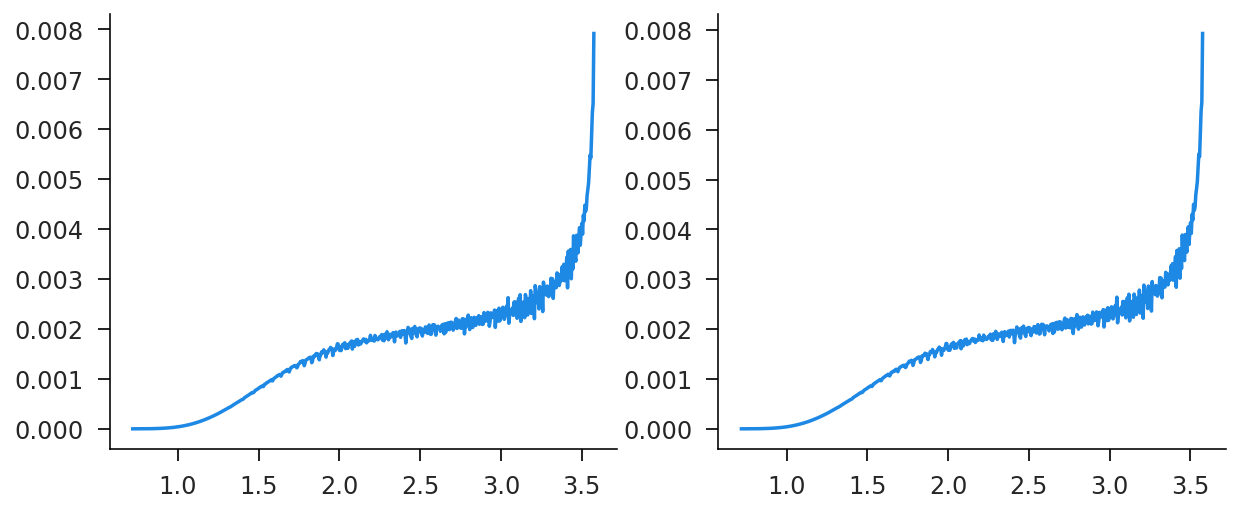### Correcting Instability¶

You can see there there are some distributional "oscillations" showing up in the previous figure. This is due to the fact that slight numerical errors get propagated as firms age. Slight imperfections in the policy function get magnified as we move up the distribution. This arises partially because firms are essentially only moving up. By adding in a bit of random Gaussian noise, we can correct this.

In :
par1 = {'γ': 0.02, **par}
vfi_fix = ps.Capital(par1, N=N)
k_fix_null = vfi_fix.fast_distribution(ret_interp['kp'], method='null')
k_fix_age = vfi_fix.fast_distribution(ret_interp['kp'], method='age')

In :
fig, (ax0, ax1) = plt.subplots(ncols=2, figsize=(10, 4))
ax0.plot(vfi_fix.k_grid, k_fix_null)
ax1.plot(vfi_fix.k_grid, k_fix_age);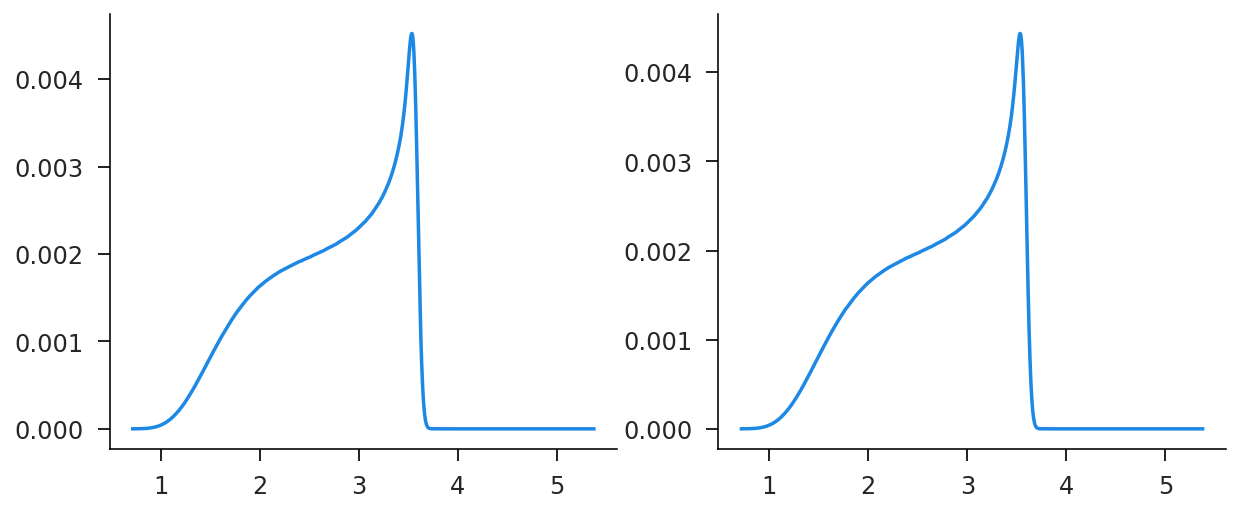In [ ]: